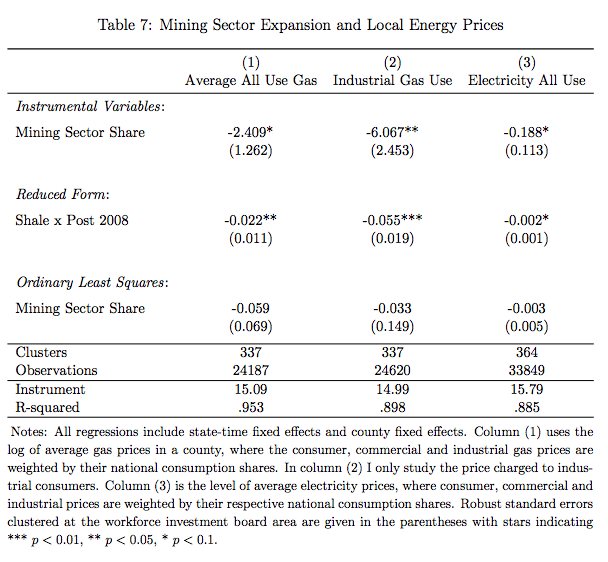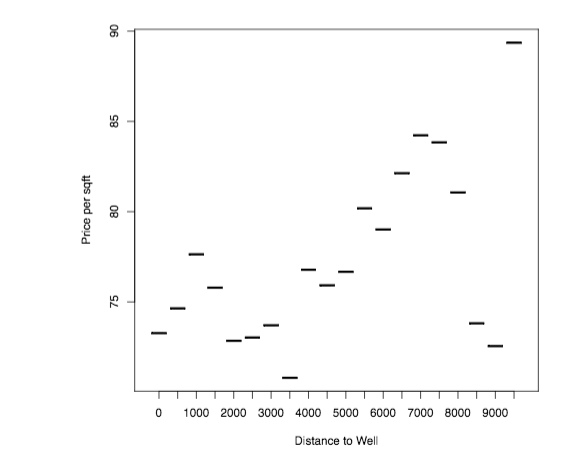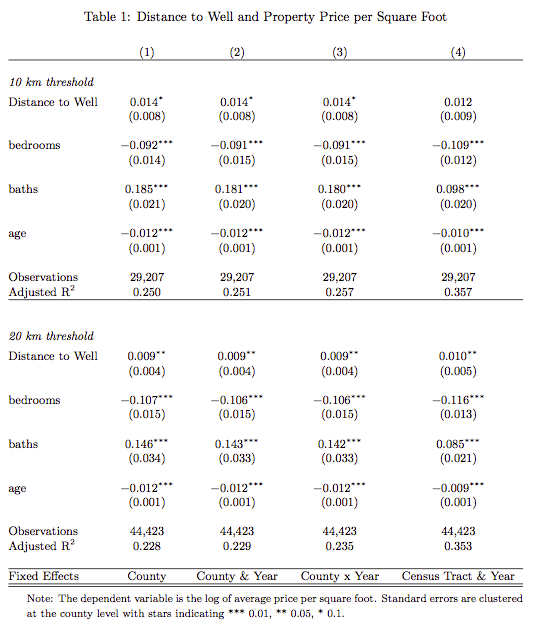# Stacking Regressions: Latex Tables with R and stargazer

In my paper on the impact of the shale oil and gas boom in the US, I run various instrumental variables specifications. For these, it is nice to stack the regression results one on the other – in particular, to have one row for the IV results, one row for the Reduced Form and maybe one row for plain OLS to see how the IV may turn coefficients around.

I found that as of now – there is no way to do that directly; please correct me if I am wrong.

The layout I have in mind is as in the screenshot of my table.Â In Stata, this can be accomplished through the use of esttab with the fragment, and append feature. This appends successive rows to an existing table and you can label the rows using the “refcat” option.

However, in R this is not possible as of yet. I have mainly worked with stargazer, as Marek has added felm objects for high dimensional fixed effects to be handled by his stargazer package.

The following functions are a “hack” that extracts the particular rows from the generated latex code by the stargazer package.

You can then piece the table together by combining the individual elements. The idea is that you have a single stargazer command that is passed to the various functions that extract the different features. Obviuously, this can be done a lot more elegant as the code is extremely hacky, but it does work

Marek has said that he is thinking of incorporating a stacking option into stargazer, but for now, my hack works reasonably well.Â The key thing to realise is that stargazer calls have the option to return

The following character strings can be used in the table.layout and omit.table.layout arguments of the stargazer command.

 “-“ single horizontal line “=” double horizontal line “-!” mandatory single horizontal line “=!” mandatory double horizontal line “l” dependent variable caption “d” dependent variable labels “m” model label “c” column labels “#” model numbers “b” object names “t” coefficient table “o” omitted coefficient indicators “a” additional lines “n” notes “s” model statistics

 

The following functions will simply extract the rows that are being returned.

stargazer.keepcolnums<-function(call="") {
command<-gsub("\\)$",", table.layout='#'\\)",command) call<-eval(parse(text=command)) bounds<-grep("tabular",call) row1<- call[(bounds+1):(bounds-1)] row1<-gsub("^\\\\\\\\\$-1\\.8ex\$\\\\hline$", "", row1)
row1<-gsub("^\\\\hline \\\\\\\\\$-1\\.8ex\$ $","",row1) row1<-row1[row1!=""] } stargazer.keeprow<-function(call="") { command<-gsub("\\)$",", table.layout='t'\\)",command)
call<-eval(parse(text=command))
bounds<-grep("tabular",call)
row1<- call[(bounds+1):(bounds-1)]
row1<-gsub("^\\\\\\\\\$-1\\.8ex\$\\\\hline $", "", row1) row1<-gsub("^\\\\hline \\\\\\\\\$-1\\.8ex\$$","",row1)
row1<-row1[row1!=""]
}

stargazer.keepstats<-function(call="") {
command<-gsub("\\)$",", table.layout='s'\\)",command) call<-eval(parse(text=command)) row1<-gsub("(.*)\\\\\\\\\$-1\\.8ex\$(.*)(\\\\end\\{tabular\\})(.*)","\\2",paste(call,collapse=" ")) row1 } stargazer.begintable<-function(call="") { command<-gsub("\\)$",", table.layout='m'\\)",command)
call<-eval(parse(text=command))
row1<-paste("\\begin{tabular}",gsub("(.*)\\\\begin\\{tabular\\}(.*)(\\\\end\\{tabular\\})(.*)","\\2",paste(call,collapse="\n")),sep="")
row1
}
stargazer.varlabels<-function(call="") {
command<-gsub("\\)$",", table.layout='d'\\)",command) call<-eval(parse(text=command)) row1<-paste("\\begin{tabular}",gsub("(.*)\\\\begin\\{tabular\\}(.*)(\\\\end\\{tabular\\})(.*)","\\2",paste(call,collapse="\n")),sep="") row1 } stargazer.keepcollabels<-function(call="") { command<-gsub("\\)$",", table.layout='c'\\)",command)
call<-eval(parse(text=command))
row1<-gsub("(.*)\\\\\\\\\$-1\\.8ex\$(.*)(\\\\end\\{tabular\\})(.*)","\\2",paste(call,collapse=" "))
row1
}

stargazer.keepomit<-function(call="") {
command<-gsub("\\)$",", table.layout='o'\\)",command) call<-eval(parse(text=command)) row1<-gsub("(.*)\\\\\\\\\$-1\\.8ex\$(.*)(\\\\end\\{tabular\\})(.*)","\\2",paste(call,collapse="\n")) row1 } It easiest to see how you can use these functions to construct stacked regression output by giving a simple example. ###the global command to be passed to the hacky ###functions that extract the individual bits ## OLS is a list of OLS results from running the ## lfe command command<-'stargazer(OLS , keep=c("post08shale:directutilityshare","post08shale"), covariate.labels=c("Energy Intensity x Shale","Shale"), header=FALSE, out.header=FALSE, keep.stat=c("n","adj.rsq"))' begintable<-stargazer.begintable(command) ###some multicolumn to combine collcombine<-c("& \\multicolumn{4}{c}{Tradable Goods Sector Only} & \\multicolumn{3}{c}{Additional Sectors} & \\cmidrule(lr){2-5} \\cmidrule(lr){6-8}") collabel<-stargazer.keepcollabels(command) colnums<-stargazer.keepcolnums(command) ##plain OLS row row1<-stargazer.keeprow(command) ##the stats part for the OLS (number of Obs, R2) stats<-stargazer.keepstats(command) ##the rows for the Fixed effect indicators omitted<-stargazer.keepomit(command) ##the IV command passing a list of IV results in ## IV object command<-'stargazer(IV , keep=c("post08anywell:directutilityshare","post08anywell"), covariate.labels=c("Energy Intensity x Anywell","Anywell"), header=FALSE, out.header=FALSE, keep.stat=c("n","adj.rsq"))' ##IV row row2<-stargazer.keeprow(command) footer<-c("\\end{tabular}\n" ) ###now combine all the items cat(begintable,collcombine,collabel,colnums,"\\hline \\\\\\emph{Reduced Form} \\\\",row1,""\\hline \\\\\\emph{Reduced Form} \\\\",row2,stats,"\\hline\\hline",footer, file="Draft/tables/energyintensity.tex", sep="\n") I know the solution is a bit hacky, but it works and does the trick. # Fracking and House Prices on the Marcellus Shale Starting last summer I worked on a short project that set out to estimate the potential costs of externalities due to unconventional shale gas production in the Marcellus shale on local house prices using a dataset of roughly 150,000 recently sold houses in Ohio, West Virginia and Pennsylvania. The data suggests that proximity to a natural gas well is correlated with lower housing prices, which confirms previous studies.I stopped working on a project that looks at the impact of nearby shale gas extraction on property prices for the Marcellus shale. Instead, I focused on my paper “Fracking Growth” that evaluates the employment consequences of the shale oil and gas boom in the US more generally. Everybody can have a look at the data and the document as it stands on sharelatex, where I also tried Sharelatex’s Knitr capacities, which are still somewhat limited as a lot of R-packages I usually work with are not yet installed. The public sharelatex file, the data and the R script can be accessed here: https://www.sharelatex.com/project/534d232b32ed2b25466b2541?r=6f73efc4&rs=ps&rm=d Here are some preliminary snippets.Â The data used in this study comes from Zillow.com In Fall 2013 I downloaded data for recently sold houses. I focused the download to cover all or most of the counties that are somewhat near the Marcellus shale in West Virginia, Ohio and Pennsylvania. This list goes back to 2011 and provides data for 151,156 sold properties. load(file = "HOUSES.rdata") library(xtable) table(HOUSES$year)
####  2011  2012  2013
#### 40087 63248 47821

A simple tabulation suggests that most data is for 2012.

Some characteristics that are included in the data are the sale price in USD, the number of bedrooms, number of bathrooms, the built up land size in square feet, the year the property was built and for some properties also the lot size.

The properties have geo-coordinates, which are used to intersect the location of the property with census-tract shapefiles. This will allow the adding of further characteristics at the census-tract level to control for general local characteristics.

The geo-coordinates are further used to compute the exact distance of a property to the nearest actual or permitted well in the year that the property was sold. Distances are computed in meters by computing the Haversine distance on a globe with radius r = 6378137 meters.

The following graph plots the average price per square foot as a function of distance to the nearest well in the year in which the property was sold. I group distances into 500 meter bins.

plot(HOUSES[, list(soldprice =sum(soldprice)/sum(sqft)), by=distancecat ], xlab="Distance to Well", ylab="Price per sqft")

A first inspection suggests a positive gradient in distance, that is – however, quite non-monotone.Non-monotonic relationship between distance to the nearest oil or gas well and price per sqft.

Does this relationship hold up when running a hedonic pricing regression?

$log(y_{ict}) = \gamma \times welldistance_{i} + \beta \times X_i + a_c + \eta_t + e_{ict}$

These are estimated using the lfe package, as I introduce quite demanding fixed effects (census-tract and county by year). The lfe package takes these fixed effects out iteratively before running the actual regression on the demeaned data.

The results for two chosen bandwidths are presented in the table below. There appears to be a positive gradient – being further away from a well correlates with higher prices per square foot.Regression results comparing sold houses nearby unconventional gas wells on the Marcellus shale

Clearly, the important question is whether one can separate out the property price appreciation that is likely to happen due to the local economic boom from the price differentials that may arise due to the presence of the local externalities and whether, one can separate out externalities due to environmental degradation as distinct from price differentials arising due to factors discussed in the beginning: no access to mortgage lending or insurances.

Unfortunately, I do not have the time to spend more time on this now, but I think a short paper is still feasible…